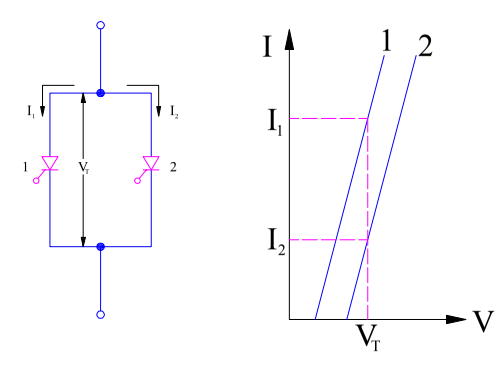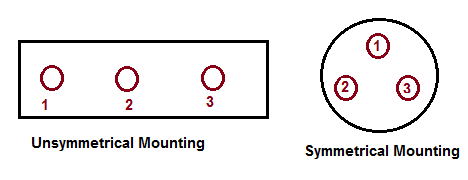# Parallel Connection of SCR and Associated Problems

Whenever the load current requirement is more than the current carrying capacity of a single SCR, two or more SCRs are connected in parallel to form a string. By having parallel connection of SCR, it is expected that individual SCR shall share load current equally and hence the string efficiency is unity. But there are various factors due to which string efficiency is less than unity. We will discuss this aspect latter in this post.

### Conditions for Parallel Connection of SCR:

Following are the necessary conditions for satisfactory parallel connection of SCR and its operation:

Let us now discuss each of the above conditions separately.

#### Effect of V-I Characteristics on Parallel Operation of SCR:

Let us consider a string of two parallel connected SCRs and their V-I characteristics as shown below.When both thyristors / SCRs are in forward conduction mode, the forward voltage drop across them will be same. Let this forward voltage drop is VT when both SCR1 and SCR2 are conducting.

Carefully observe the V-I characteristics of SCR1 and SCR2. It can be seen that for voltage VT the current flowing through SCR1 is I1 whereas that for SCR2 is I2. I2 is very small as compared to I1. This means, the current shared by the SCRs are not equal. SCR1 is carrying more current I1 whereas SCR2 is carrying lesser current I2. Ideally both the SCRs should carry current equal to I1 but the unequal sharing of current arises just due to difference in their V-I characteristics.

Therefore the total load current will be (I1+I2) instead of 2I1. Thus the string efficiency for this parallel connection SCR will be equal to

String Efficiency

= (I1+I2) / 2I1

= 0.5[1+(I2/I1)]

Thus we see that, string efficiency is less than unity because of difference in V-I characteristics of SCR in parallel connected in string.

#### Effect of Dynamic Characteristics on Parallel Operation of SCR:

Different dynamic characteristics of SCRs will result in different run-on and turn-off time. Let us assume that the turn-on time of SCR1 is more than that of SCR2. When the SCRs are fired or gated simultaneously, SCR2 will turn-on earlier. What will happen then?

Since SCR2 is conducting, the voltage drop across it will drop to VT. As SCR1 and SCR2 are connected in parallel, the voltage across them will be equal. This means, the voltage across SCR2 will become VT once SCR2 starts conducting. Since VT is vey less, SCR1 may not trun-on even though it is gated. This is because, there is a term called finger voltage which is minimum voltage across SCR which must exist for bringing it in forward conduction mode when gated. Since VT may be less than finger voltage, it may not come in forward conduction mode even after gated. So think, why did this happen? This happened just because the turn-on time of SCR1 was more than that of SCR2.  In such case, SCR2 will share the entire current may get overheated and may lead to subsequent damage.

#### Effect of Different Temperature of Parallel Connected SCR:

If once SCR1 in parallel unit carry more current than other SCRs, then this SCR will have more junction temperature. Agree? As a result, its dynamic resistance will decrease. This decrease in dynamic resistance will cause increase in current flowing through this SCR1.  This will again increase the junction temperature and subsequent reduction in dynamic resistance. This reduction is dynamic resistance will further increase the current through SCR1. Thus, this process is cumulative and will eventually lead to damage of SCR1 due to excessive junction temperature. After damaging of SCR1, this phenomena may engulf other SCRs connected in parallel string may destroy them.

Thus it is very important that all the SCR connected in parallel should be operated that equal temperature. To do this, we should mount them on the same heat sink.

#### Effect of Mounting of SCRs on Heat Sink:

Unequal current sharing in parallel connected SCR may also arise due to unsymmetrical mounting on heat sink. Let us understand this using figure shown below.In unsymmetrical mounting, the middle conductor 2 will have maximum magnetic flux linkage due to presence of two nearby conductors 1 & 3. This will cause conductor 2 to have maximum inductance among the three conductors. Therefore, the current flowing through middle conductor 2 will be lower as compared to remaining conductor 1 & 3. This problem of unequal current sharing due to unsymmetrical spacing can easily be resolved by mounting all the SCRs symmetrically. The symmetrical mounting will cause equal flux linkage of all the conductors 1, 2 & 3 and therefore equal inductance. Therefore, the current flow in all the SCRs will be equal.

This site uses Akismet to reduce spam. Learn how your comment data is processed.